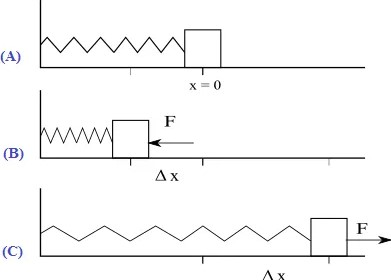# Set up differential equation for an oscillation of a spring using Hooke’s and Newton’s second law. Find the general solution of this equation and hence the expressions for the period, velocity and oscillation of the acceleration

Consider a mass less spring having spring constant ‘k’, one end of which is connected to a mass ‘m’ and other is connected to a fixed support.

The mass ‘m’ is free to move on a friction less horizontal surface. The equilibrium position is shown in 1(a) as relaxed and no force is acting on it. If a force is applied on the mass to stretch and then released the motion of the mass is simple harmonic.Hooke’s law states that the extension or compression x of an elastic body is proportional to the applied force F.

F ∝ x

F = -kx …………. (1)

Here, -ve sign shows that F and x are in opposite direction where k is constant of proportionality called force constant or spring constant. The value of k characterizes the strength of the spring. A spring with a large k is stronger or stiffer than with a small k.

By Newton’s 2nd law,

$$F = ma = m \frac{dx^2}{dt^2}$$ ……..(2)

Comparing equations (1) and (2)

$$\Rightarrow m \frac{d^{2}x}{dt^2} = -kx$$

$$\Rightarrow m \frac{d^{2}x}{dt^2} + kx = 0$$

$$\Rightarrow \frac{d^{2}x}{dt^2} + \frac{k}{m}x = 0$$

i.e $$\frac{d^{2}x}{dt^2}$$ + ω2x = 0 ……… (1)

This is the equation of SHM,

where, ω2 = $$\frac{k}{m}$$

ω = $$\sqrt{\frac{k}{m}}$$

$$T = \frac{2 \pi}{ω} = \frac{2\pi \sqrt{m}}{k}$$

The solution of equation (1) is given by, x = xmsin(ωt + Ø)

Velocity

We have, x = xmsin(ωt + Ø)

velocity, v = $$\frac{dx}{dt}$$ = ωxmcos(ωt + Ø) => vmax = xmω

$$v = \omega x_{m}\sqrt{1-sin^{2}(\omega t – \Theta)}$$

$$= \omega x_{m} \sqrt{1 – \frac{x^2}{x_m^2}}$$

$$= \omega x_{m} \sqrt{\frac{x_m^2 – x^2}{x_m^2}}$$

$$= \omega \sqrt{A^2 – x^2}$$  (where xm = A )

Acceleration

We have, v = ωxmcos(ωt + Ø)

Acceleration, a = $$\frac{dv}{dt}$$

= -ω2xmsin(ωt + Ø) => amax = -ω2xm

∴ a = -ω2x

where -ve sign shows ‘a’ is directed towards mean position.

Time Period

The time taken by particle to complete a cycle of oscillation is called time period ‘T’.

This means the displacement ‘x’ returns to its initial value after one complete oscillation i.e in time period T and the sine function repeat in angle 2π.

Therefore, x = xmsinω(t + T) = xmsin(ωt + 2π).

Frequency

The number of complete oscillation made by an oscillation particle in one second is called Frequency. It is denoted by,

f = 1/T

Here, T is time required for one complete oscillation is called time period.

The angular speed ω is related to the frequency of rotation f rather simply. By definition, ω is the change in angular displacement per unit time.

ω = θ/t

For t = T (time period),  θ = 2π

Therefore, ω = $$\frac{2\pi}{T}$$ = 2πf.